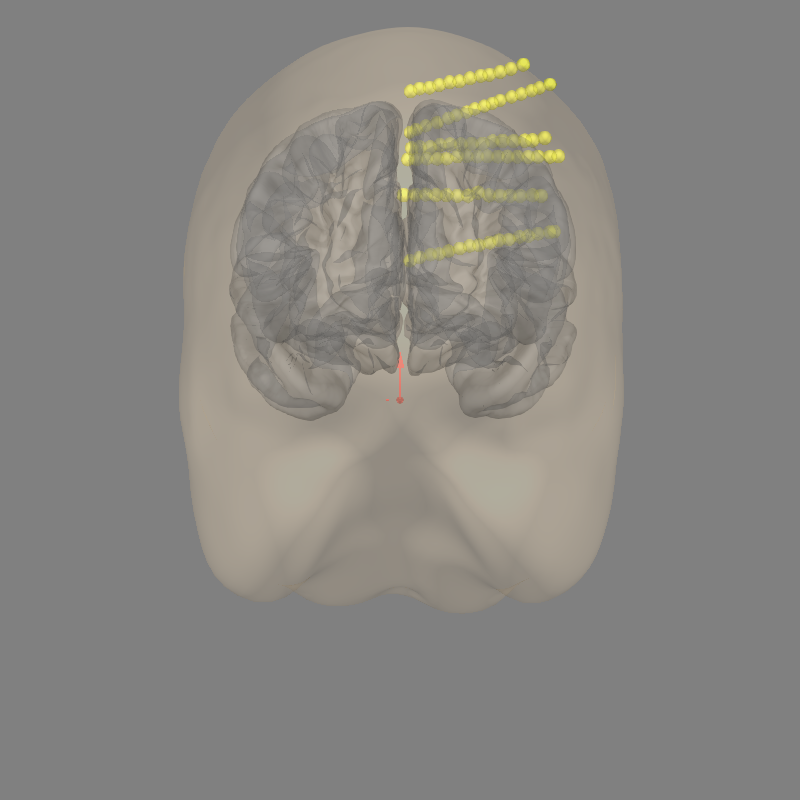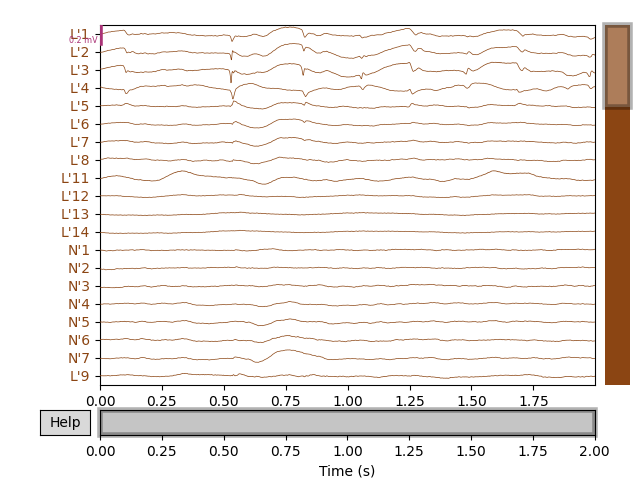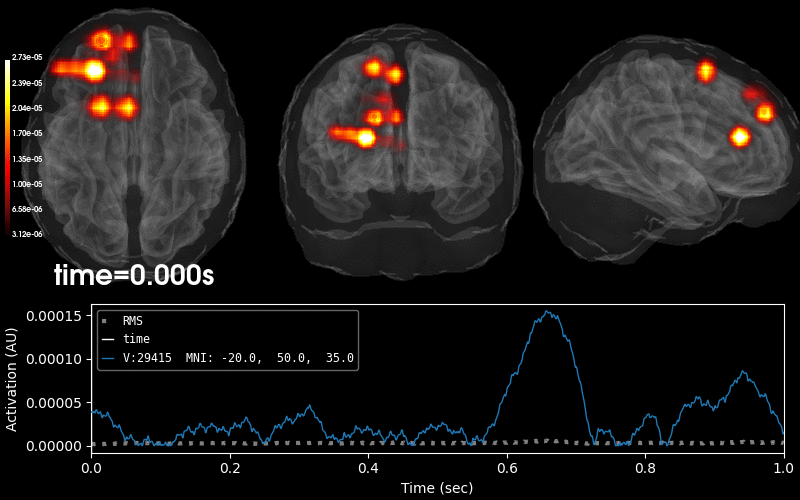# Working with sEEG data¶

MNE supports working with more than just MEG and EEG data. Here we show some of the functions that can be used to facilitate working with stereoelectroencephalography (sEEG) data.

This example shows how to use:

• sEEG data

• channel locations in MNI space

• projection into a volume

Note that our sample sEEG electrodes are already assumed to be in MNI space. If you want to map positions from your subject MRI space to MNI fsaverage space, you must apply the FreeSurfer’s talairach.xfm transform for your dataset. You can take a look at How MNE uses FreeSurfer’s outputs for more information.

For an example that involves ECoG data, channel locations in a subject-specific MRI, or projection into a surface, see Working with ECoG data. In the ECoG example, we show how to visualize surface grid channels on the brain.

```# Authors: Eric Larson <larson.eric.d@gmail.com>
#

import os.path as op

import numpy as np
import pandas as pd

import mne
from mne.datasets import fetch_fsaverage

print(__doc__)

# paths to mne datasets - sample sEEG and FreeSurfer's fsaverage subject
# which is in MNI space
misc_path = mne.datasets.misc.data_path()
sample_path = mne.datasets.sample.data_path()
subject = 'fsaverage'
subjects_dir = sample_path + '/subjects'

# use mne-python's fsaverage data
```

Out:

```0 files missing from root.txt in /home/circleci/mne_data/MNE-sample-data/subjects
0 files missing from bem.txt in /home/circleci/mne_data/MNE-sample-data/subjects/fsaverage
```

Let’s load some sEEG electrode locations and names, and turn them into a `mne.channels.DigMontage` class. First, use pandas to read in the `.tsv` file.

```# In mne-python, the electrode coordinates are required to be in meters
ch_names = elec_df['name'].tolist()
ch_coords = elec_df[['x', 'y', 'z']].to_numpy(dtype=float)

# the test channel coordinates were in mm, so we convert them to meters
ch_coords = ch_coords / 1000.

# create dictionary of channels and their xyz coordinates (now in MNI space)
ch_pos = dict(zip(ch_names, ch_coords))

# Ideally the nasion/LPA/RPA will also be present from the digitization, here
# we use fiducials estimated from the subject's FreeSurfer MNI transformation:
lpa, nasion, rpa = mne.coreg.get_mni_fiducials(
subject, subjects_dir=subjects_dir)
lpa, nasion, rpa = lpa['r'], nasion['r'], rpa['r']
```

Now we make a `mne.channels.DigMontage` stating that the sEEG contacts are in the FreeSurfer surface RAS (i.e., MRI) coordinate system for the given subject. Keep in mind that `fsaverage` is special in that it is already in MNI space.

```montage = mne.channels.make_dig_montage(
ch_pos, coord_frame='mri', nasion=nasion, lpa=lpa, rpa=rpa)
print('Created %s channel positions' % len(ch_names))
```

Out:

```Created 88 channel positions
```

Now we get the trans that transforms from our MRI coordinate system to the head coordinate frame. This transform will be applied to the data when applying the montage so that standard plotting functions like `mne.viz.plot_evoked_topomap()` will be aligned properly.

```trans = mne.channels.compute_native_head_t(montage)
print(trans)
```

Out:

```<Transform | MRI (surface RAS)->head>
[[ 9.99993682e-01  3.55187384e-03  2.02048104e-04 -1.76272495e-03]
[-3.55756865e-03  9.98389125e-01  5.66258579e-02  3.10943504e-02]
[-5.94736775e-07 -5.66262193e-02  9.98395443e-01  3.95972491e-02]
[ 0.00000000e+00  0.00000000e+00  0.00000000e+00  1.00000000e+00]]
```

Now that we have our montage, we can load in our corresponding time-series data and set the montage to the raw data.

```# first we'll load in the sample dataset

raw.info['bads'].extend([ch for ch in raw.ch_names if ch not in ch_names])
raw.crop(0, 2)  # just process 2 sec of data for speed

# attach montage
raw.set_montage(montage)

# set channel types to sEEG (instead of EEG) that have actual positions
raw.set_channel_types(
{ch_name: 'seeg' if np.isfinite(ch_pos[ch_name]).all() else 'misc'
for ch_name in raw.ch_names})
```

Out:

```Extracting EDF parameters from /home/circleci/mne_data/MNE-misc-data/seeg/sample_seeg.edf...
EDF file detected
Setting channel info structure...
Creating raw.info structure...
Reading 0 ... 19999  =      0.000 ...    19.999 secs...
```
Measurement date January 01, 2000 12:01:01 GMT Unknown Unknown 91 points 0 magnetometer, 0 gradiometer, and 0 EEG channels Not available Not available 1000.00 Hz 0.00 Hz 500.00 Hz sample_seeg.edf 00:00:02 (HH:MM:SS)

Let’s check to make sure everything is aligned.

```fig = mne.viz.plot_alignment(raw.info, trans, 'fsaverage',
subjects_dir=subjects_dir, show_axes=True,
```Out:

```Using outer_skin.surf for head surface.
Plotting 88 sEEG locations
```

Next, we’ll get the raw data and plot its amplitude over time.

```raw.plot()
```We can visualize this raw data on the `fsaverage` brain (in MNI space) as a heatmap. This works by first creating an `Evoked` data structure from the data of interest (in this example, it is just the raw LFP). Then one should generate a `stc` data structure, which will be able to visualize source activity on the brain in various different formats.

```# get standard fsaverage volume (5mm grid) source space
fname_src = op.join(subjects_dir, 'fsaverage', 'bem',
'fsaverage-vol-5-src.fif')

evoked = mne.EvokedArray(raw.get_data(), raw.info).crop(0, 1)  # shorter
stc = mne.stc_near_sensors(
evoked, trans, subject, subjects_dir=subjects_dir, src=vol_src,
verbose='error')  # ignore missing electrode warnings
stc = abs(stc)  # just look at magnitude
clim = dict(kind='value', lims=np.percentile(abs(evoked.data), [10, 50, 75]))
```

Out:

```Reading a source space...
[done]
```

Plot 3D source (brain region) visualization:

By default, `stc.plot_3d()` will show a time course of the source with the largest absolute value across any time point. In this example, it is simply the source with the largest raw signal value. Its location is marked on the brain by a small blue sphere.

```brain = stc.plot_3d(
src=vol_src, subjects_dir=subjects_dir,
view_layout='horizontal', views=['axial', 'coronal', 'sagittal'],
size=(800, 300), show_traces=0.4, clim=clim,